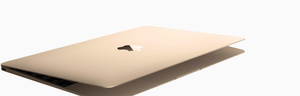# Refurbished Mac

Discover what goes into each refurbished Mac.A\$849.00

A\$1,039.00

A\$1,099.00

A\$1,099.00

A\$1,099.00

A\$1,359.00

A\$1,359.00

A\$1,359.00

A\$1,359.00

A\$1,489.00

A\$1,529.00

A\$1,529.00

A\$1,529.00

A\$1,529.00

A\$1,609.00

A\$1,609.00

A\$1,609.00

A\$1,679.00

A\$1,699.00

A\$1,709.00

A\$1,709.00

A\$1,869.00

A\$1,869.00

A\$1,869.00

A\$1,869.00

A\$1,869.00

A\$1,949.00

A\$1,949.00

A\$1,949.00

A\$1,949.00

A\$1,969.00

A\$1,969.00

A\$1,969.00

A\$2,059.00

A\$2,069.00

A\$2,119.00

A\$2,119.00

A\$2,119.00

A\$2,119.00

A\$2,119.00

A\$2,189.00

A\$2,209.00

A\$2,219.00

A\$2,219.00

A\$2,299.00

A\$2,339.00

A\$2,379.00

A\$2,379.00

A\$2,379.00

A\$2,459.00

A\$2,459.00

A\$2,469.00

A\$2,469.00

A\$2,469.00

A\$2,509.00

A\$2,519.00

A\$2,609.00

A\$2,629.00

A\$2,629.00

A\$2,629.00

A\$2,719.00

A\$2,719.00

A\$2,739.00

A\$2,739.00

A\$2,739.00

A\$2,789.00

A\$2,849.00

A\$2,879.00

A\$2,879.00

A\$2,879.00

A\$2,879.00

A\$3,009.00

A\$3,009.00

A\$3,009.00

A\$3,059.00

A\$3,109.00

A\$3,109.00

A\$3,249.00

A\$3,279.00

A\$3,279.00

A\$3,329.00

A\$3,399.00

A\$3,399.00

A\$3,399.00

A\$3,399.00

A\$3,569.00

A\$3,649.00

A\$3,649.00

A\$3,869.00

A\$3,909.00

A\$4,029.00

A\$4,119.00

A\$4,139.00

A\$4,249.00

A\$4,249.00

A\$4,269.00

A\$4,379.00

A\$4,379.00

A\$4,529.00

A\$4,719.00

A\$4,719.00

A\$4,759.00

A\$4,759.00

A\$4,959.00

A\$4,959.00

A\$5,049.00

A\$5,139.00

A\$5,399.00

A\$5,399.00

A\$5,649.00

A\$5,649.00

A\$5,759.00

A\$6,129.00

A\$6,569.00

A\$6,669.00

A\$6,669.00

A\$6,799.00

A\$6,799.00

A\$7,039.00

A\$7,039.00

A\$7,439.00

A\$7,479.00

A\$7,479.00

A\$7,479.00

A\$8,019.00